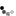# Bipolar Junction Transistor (BJT) Switch

The Bipolar Junction Transistor can be used as a switch. This is required when the typical digital output (max current output 20ma) does not provide sufficent current drive for high current devices like lamps, solenoids or motors. The transistor current gain provides the order of magnitude increase in collector current.First we redraw the schematic using the BJT DC equivalent circuit

Using KCL (Kirchhoff's current law)
Current through RB \begin{equation} I_{RB} = I_B \end{equation} Current through RC \begin{equation} \label{1} I_C = \beta I_B \end{equation} Current at Node E \begin{equation} I_E = I_B + I_C \end{equation}

Using KVL (Kirchhoff's voltage law) \begin{equation} V_B = I_BR_B + V_{BE} \end{equation} \begin{equation} \label{2} V_C = I_CR_C + V_{CE} \end{equation} Substitute equation \ref{1} into equation \ref{2}: \begin{equation} \label{3} V_C = \beta I_BR_C + V_{CE} \end{equation}

If after substituting circuit values into the equations and we obtain VCE <0 in equation \ref{3} , then the transistor behaves like an on-switch. It is no longer a current controlled current source, and equations \ref{4} and \ref{5} apply instead. \begin{equation} \label{4} V_{CE}=0 \end{equation} Equation \ref{2} simplifies to equation \ref{5} and IC is no longer dependent on IB \begin{equation} \label{5} V_C = I_CR_C \end{equation}

R1 R2Notes

• Observe the node voltages when you change VIN by adjusting the knob.
• To calculate the node voltages
1. Use equation 4 to calculate IB. Assume VBE=0.7
2. When VIN<0.7V, assume IB=0. See diode circuit analysis for explanation.
3. Assume β=100, and use equation 6 to calculate VCE
• Change the values of R1 and R2 and press the Simulate button. If you have a small load (R2=100 ohm), you will need a sufficiently large base current to operate the BJT as a switch (observe the problem when R1=10K). One way to reduce this base current is to use a Darlington transistor.
• In a real transistor VCE never reaches 0, instead it saturates at around 0.1V. This is also known as the saturation voltage of a BJT. In this operation the collector current IC is determined using equation 8.